• 文章目录机器学习中常见的概率分布1.Bernoulli分布（伯努利分布）2.Multinoulli分布（多项式分布的一个特例）3.高斯分布4.指数分布和Laplace分布（拉普拉斯分布）5.Dirac分布（狄拉克分布）和经验分布 机器学习...
文章目录机器学习中常见的概率分布1.Bernoulli分布（伯努利分布）2.Multinoulli分布（多项式分布的一个特例）3.高斯分布4.指数分布和Laplace分布（拉普拉斯分布）5.Dirac分布（狄拉克分布）和经验分布
机器学习中常见的概率分布
在机器学习中有许多简单的概率分布，掌握这些概率分布有助于思考问题。
1.Bernoulli分布（伯努利分布）
Bernoulli分布是单个二值随机变量的分布。一个简单的实验只有两个可能的结果，例如抛硬币的正面和反面、做一件事成功或失败，将这两种情况记作0和1，即随机变量只能取值为0和1，并由单个参数$\phi$给出随机变量等于1的概率。
Bernoulli分布具有一些性质。
$P(X=1) = \phi \\ P(X=0) = 1-\phi$
概率质量函数为：
$P(X=x)=\phi^x(1-\phi)^{1-x}$
对于$f(x)=x$，期望和方差为：
$E_x[f(x)]=1*\phi+0*(1-\phi)=\phi \\ Var_x[f(x)]=E[(f(x)-E[f(x)])^2]=\phi(1-\phi)$
2.Multinoulli分布（多项式分布的一个特例）
Multinoulli分布也叫范畴分布（categorical distribution），是Bernoulli分布的泛化，如果说Bernoulli分布代表着一个只有两种结果的简单实验，那Multinoulli分布就是可能有$k$个结果的实验。随机向量$X$定义为：
$X=[X_1, X_2,\cdots, X_k]$
当得到第$i$个结果时，随机向量$X$的第$i$个值即$X_i$为1，其他为0。$k$个可能的结果的概率则用$p_1, p_2,\cdots,p_K$来表示。
$X$是$K×1$的离散随机向量，$R_X$为$X$的支持，其中一个量为1，其他量均为0：
$R_X=\{x\in\{0,1\}^K:\sum_{j=1}^Kx_j=1\}$
$p_1, p_2, \cdots, p_K$为$K$个非负数，并且满足：
$\sum_{j=1}^Kp_j=1$
这时，如果有如下联合概率质量函数我们就说$X$有一个Multinoulli分布并且概率为$p_1,p_2,\cdots,p_K$。
$p_X(x_1, x_2,\cdots,x_K)= \begin{cases} \prod_{j=1}^Kp_j^{x_j} &if(x_1,x_2,\cdots,x_K)\in R_X \\ 0 &otherwise \end{cases}$
当$(x_1,x_2,\cdots,x_K)\in R_X$并且此时有$x_i=1$，这说明其他的值都是0，因此就有下面的式子：
$\prod_{j=1}^Kp_j^{x_j}=p_1^{x_1}*\cdots *p_i^{x_i}*\cdots *p_K^{x_K} \\ =p_1^{0}*\cdots *p_i^{1}*\cdots *p_K^{0} \\=1*\cdots *p_i*\cdots*1 \\=p_i$
Multinoulli分布经常用来表示对象分类的分布，所以我们很少假设状态1具有数值1之类的。因此，我们通常不需要去计算Multinoulli分布的随机变量的期望和方差。
3.高斯分布
实数上最常用的分布就是正态分布，也称高斯分布。若随机变量X服从一个数学期望为$\mu$、方差为$\sigma^2$的正态分布，记为$N(\mu，\sigma^2)$。概率密度函数为
$N(x:\mu,\sigma^2)=\sqrt{\cfrac{1}{2\pi\sigma^2}}exp(-\cfrac{1}{2\sigma^2}(x-\mu)^2)$
其概率密度函数为正态分布的期望值$\mu$决定了其位置，其标准差$\sigma$决定了分布的幅度。当$\mu = 0,\sigma = 1$时的正态分布是标准正态分布。
正态曲线呈钟型，两头低，中间高，左右对称因其曲线呈钟形，因此人们又经常称之为钟形曲线。
当我们要对概率密度函数求值时，需要对$\sigma$平方并取倒数。当我们需要经常对不同参数下的概率密度函数求值时，一种更高效的参数化分布的方式是使用参数$\beta\in(0,\infty)$来控制分布的精度（或方差的倒数）：
$N(x:\mu,\beta^{-1})=\sqrt{\cfrac{\beta}{2\pi}}exp(-\cfrac{1}{2}\beta(x-\mu)^2)$
进一步，正态分布可以推广到$R_n$空间，这种情况下被称为多维正态分布。它的参数是一个正定对称矩阵$\Sigma$：
$N(x:\mu,\Sigma)=\sqrt{\cfrac{1}{(2\pi)^n det(\Sigma)}}exp(-\cfrac{1}{2}(x-\mu)^T\Sigma^{-1}(x-\mu))$
这式子看起来很复杂的样子，我只是简单了解了一下。参数$\mu$仍然表示分布的均值，只不过现在是向量值。参数$\Sigma$给出了分布的协方差矩阵。和单变量的情况类似，当我们希望对很多不同参数下的概率密度函数多次求值时，协方差矩阵并不是一个很高效的参数化分布方式，因为对概率密度函数求值时需要对$\Sigma$求逆。我们可以使用一个精度矩阵$\beta$进行替代：
$N(x:\mu,\beta^{-1})=\sqrt{\cfrac{det(\beta)}{(2\pi)^n}}exp(-\cfrac{1}{2}(x-\mu)^T\beta(x-\mu))$
我们常常把协方差矩阵固定成一个对角阵。一个更简单的版本是各向同性高斯分布，它的协方差矩阵是一个标量乘以单位阵。
4.指数分布和Laplace分布（拉普拉斯分布）
深度学习中，我们经常会需要一个在$x=0$点处取得边界点的分布。为了实现这一目的，我们可以使用指数分布：
$p(x;\lambda)=\lambda1_{x\ge0}exp(-\lambda x)$
其中用指示函数$1_{x\ge0}$来使得当$x$取负值时的概率为0。
一个联系紧密的概率分布是Laplace分布，它允许我们在任意一点$\mu$处设置概率质量的峰值：
$Laplace(x;\mu, \gamma)=\cfrac{1}{2\gamma}exp(-\cfrac{|x-\mu|}{\gamma})$
其中$\mu$是位置参数，$\gamma$是尺度参数，果$\mu = 0$，那么，正半部分恰好是尺度为$\cfrac{1}{\gamma}$（或者$\gamma$，看具体指数分布的尺度参数形式）的指数分布的一半。
5.Dirac分布（狄拉克分布）和经验分布
在一些情况下，我们希望概率分布中的所有质量都集中在一个点上。这可以通过Dirac delta函数$\delta(x)$定义概率密度函数来实现：
$p(x)=\delta(x-\mu)$
$\delta(x)$是一个广义函数，在物理学中常用其表示质点、点电荷等理想模型的密度分布，该函数在除了零以外的点取值都等于零，而其在整个定义域上的积分等于1。通过把$p(x)$定义成$\delta$函数右移$\mu$个单位，我们就得到了一个在$x=\mu$处具有无限窄也无限高的概率质量。
Dirac分布经常作为经验分布的一个组成部分出现：
$\hat p(x)=\cfrac{1}{m}\sum_{i=1}^m\delta(x-x^{(i)})$
经验分布将概率密度$\cfrac{1}{m}$赋给$m$个点的每一个，这些点是给定数据集或者采样的集合。只有在定义连续型随机变量的经验分布时，$\delta(x)$函数才是必要的。对于离散型随机变量，情况更加简单：经验分布可以被定义成一个Multinoulli分布，对于每一个可能的输入，其概率可以简单地设为在训练集上哪个输入值的经验概率。
当我们在训练集上训练模型时，可以认为从这个训练集上得到的经验分布指明了采样来源的分布。关于经验分布另外一种重要的观点是，它是训练数据的似然最大的那个概率密度函数。
参考资料《深度学习》


展开全文• 本文介绍通信专业常用到一些概率分布
几个重要的分布
Gaussian Distribution

standard Gaussian distribution
$X\sim \mathcal N(0,1)$
$f(x)=\dfrac{1}{\sqrt{2\pi}}e^{-\dfrac{x^2}{2}}$
$\Phi(x)=\dfrac{1}{\sqrt{2\pi}}\int_{-\infty}^{x}e^{-\dfrac{t^2}{2}}dt​$

general Gaussian distribution
$X\sim \mathcal N(\mu,\sigma^2)$
$f(x)=\dfrac{1}{\sqrt{2\pi}\sigma}e^{-\dfrac{(x-\mu)^2}{2\sigma^2}}$
$F(x)=\dfrac{1}{\sqrt{2\pi}\sigma}\int_{-\infty}^{x}e^{-\dfrac{(t-\mu)^2}{2\sigma^2}}dt$

several functions related to Gaussian distribution
$Q$函数定义为标准正态分布的右尾函数：
$Q(x)=\frac{1}{\sqrt{2\pi}}\int_x^\infty e^{-\tfrac{t^2}{2}}dt = 1-\Phi(x)$
误差函数 $erf(x)$ 的物理意义是服从均值为$0$, 方差为$\frac{1}{2}$正态分布的随机变量$Y$落在区间$(-x, x)$的概率：
$erf(x)=\frac{2}{\sqrt{\pi}}\int_0^xe^{t^2}dt$
互补误差函数$erfc(x)$的物理意义即$Y$落在上述区间外的概率：
$erfc(x)=\frac{2}{\sqrt{\pi}}\int_x^{\infty}e^{t^2}dt$
显然：
$erfc(x)=1-erfc(x)$
$Q(x) = \frac{1}{2}erfc(\frac{x}{\sqrt{2}})$

Chi-Square Distribution

definition
设$X_1, X_2, ..., X_n$ i.i.d. $\sim \mathcal N(0,1)$，令$X=\sum_{i=1}^nX_i^2$，则称$X$服从自由度为$n$的 Chi-Square distribution $\chi^2(n)$

probability density function
$f_n(x)=\dfrac{(1/2)^{n/2}}{\Gamma(n/2)}x^{n/2-1}e^{-x/2}$
上式中$k$表示自由度，$\Gamma(·)$函数是阶乘函数的推广，$\Gamma(n)=(n-1)！$。当$n=2$时
$f(x)=\frac{1}{2}e^{-x/2}$
i.e. Chi-Square distribution 退化为均值为2的指数分布。

mean = $n$ and variance = $2n$

exponential distribution with mean $\lambda$ and variance $\lambda^2$ has PDF:
$f(x)=\frac{1}{\lambda}e^{-\frac{x}{\lambda}}$

Rayleigh Distribution
$R\sim \rm{Rayleigh}(\sigma)$ if $R=\sqrt{X^2+Y^2}$，where $X\sim N(0, \sigma^2)$ and $Y\sim N(0, \sigma^2)$ are independent normal random variables.
$f(r)=\frac{r}{\sigma^2}e^{-\frac{r^2}{2\sigma^2}}$

mean and variance are proportional to $\sigma$ and $\sigma^2$ respectively.

One example where the Rayleigh distribution naturally arises is when wind velocity is analyzed in two dimensions. Assuming that each component is uncorrelated, normally distributed with equal variance, and zero mean, then the overall wind speed (vector magnitude) will be characterized by a Rayleigh distribution.
A second example of the distribution arises in the case of random complex numbers whose real and imaginary components are independently and identically distributed Gaussian with equal variance and zero mean. In that case, the absolute value of the complex number is Rayleigh-distributed.
The Chi-Square distribution with $n = 2$ is equivalent to the Rayleigh Distribution with $\sigma = 1$. I.e., if ${R\sim \mathrm {Rayleigh} (1)}$, then $R^2\sim \chi^2(2)$. I have mentioned above that Chi-Square distribution with $n=2$ is the same as exponential distribution with mean 2, so there must be a relationship between Rayleigh distribution and exponential distribution:
$X\sim E(\lambda)\\ \frac{X}{\lambda/2}\sim E(2)\\ \frac{X}{\lambda/2}\sim \chi^2(2)\\ \sqrt{\frac{X}{\lambda/2}}\sim \rm{Rayleigh}(1)\\ \sqrt{X}\sim \rm{Rayleigh}(\sqrt{\lambda/2})$
Consider fading coefficient $h\sim\mathcal{CN}(0, 1)$, we know that $|h|$ is Rayleigh distributed with parameter $\sigma^2=\frac{1}{2}$ (each dimension). So the squared magnitude $r=|h|^2$ is exponential distributed with mean $2\sigma^2=1$, i.e.
$f(r)=e^{-r}$


展开全文数字通信
• 本文介绍一些常见的概率论知识和概率分布
Introduction
2019年3月更新：最近略忙，不，是超忙，更的会慢些，我先把《神经网络与深度学习》翻译完。
最近看了一些有关网络和图的文章，遇到一些陌生的概率分布，学习之后，在这里一并描述，持续更新，文末配有 Python Matplotlib 代码，用语通俗，错误难免，还请读者斧正，函数具体如下：
IndexProbability Density Function (PDF)，概率密度函数Bimodal DistributionCumulative Distribution Function (CDF)，累计分布函数Complementary Cumulative Distribution Function (CCDF)，互补累积分布函数Quantile Funtion (PPF)，分位函数Dirac Delta Function，狄拉克 δ 函数Exponential Distribution，指数分布Heavy-tailed Distribution 重尾分布Long-tailed Distribution，长尾分布Subexponential Distribution，次指数分布Fat-tailed Distribution，肥尾分布Log-normalPareto DistributionMarginal DistributionMarginal Joint DistributionMultimodal DistributionBimodal DistributionMultinomial DistributionPower-lawUnimodal DistributionWeibull DistributionZipfian Distribution (Zipf's law)
Preliminaries

我将用一个微博转发数据集  贯穿本文来说明一些分布的特性，数据集包含119,313条微博，每条微博最少被转发过10次，其中包含的信息有哪些人转发了这条微博，以及每次转发的时间。
Probability Density Function (PDF)，概率密度函数
Bimodal Distribution
Cumulative Distribution Function (CDF)，累计分布函数

定义：
$F_X(x) = P(X \le x)$
两个关键点，一个 $X$， 一个 $x$，前者代表随机变量，后者代表一个实值。
举个例子，掷骰子，可能出现的结果 $X \in \{1, 2, 3, 4, 5, 6\}$， $P(X) = 1/6$，假如我们让 $x = 6$，投一次骰子，结果小于等于 $x$ 的概率是多少呢？答案是1。如果取 $x = 0$， 因为不可能投出小于等于零的骰子，所以概率 $P(X \le 0) = 0$。
这个简单的例子表明，累积分布函数在 $x \rarr -\infty$ 时等于 $0$，在 $x \rarr \infty$ 时等于 $1$，而且是非减、右连续的。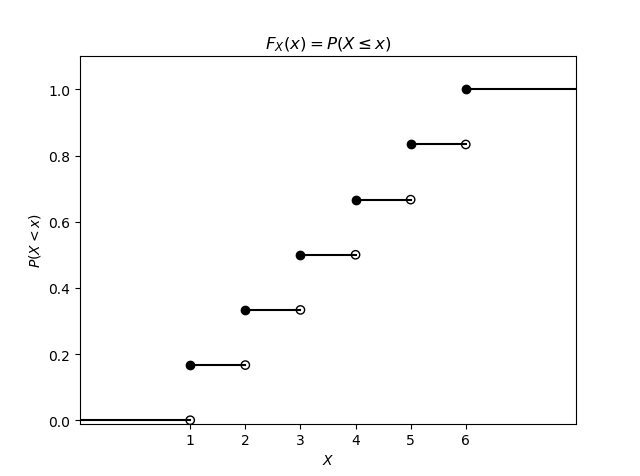如图所示，给定任意一个 $X$，例如 $3$，可知投的骰子的数小于等于 $3$ 的概率为 $0.5$。
再用微博举一个例子，微博转发数满足下面这个累积分布：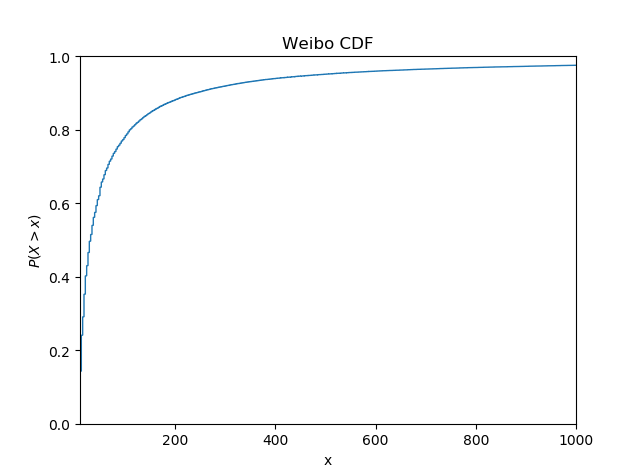对于一条微博，它转发数小于某个 $x$ 的概率是多少，可以很方便地在图中看出来。
假如现在有一正态分布 $X \sim N(1.7, 0.2^2)$ 表示一个班级内50个学生的身高分布，其累积分布为：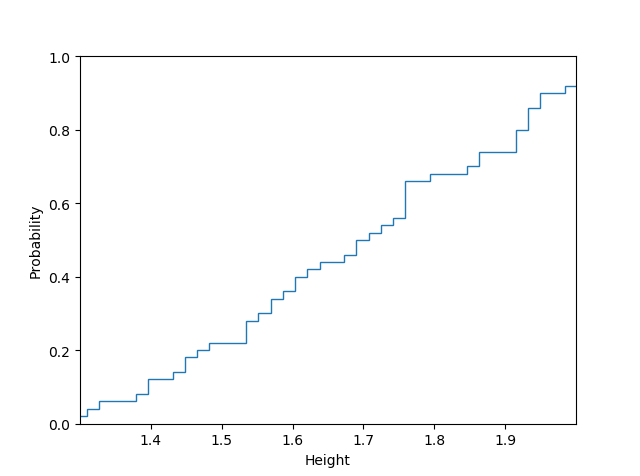由图可知，身高低于190厘米的概率大约是 $0.75$，显然，正态分布的标准差设的有点大了。
Complementary Cumulative Distribution Function (CCDF)，互补累积分布函数

定义：
$\bar{F}_X(x) = P(X > x) = 1 - F_X(x)$
定义很简单，用 $1$ 减去原始的累积分布函数 $F_X(x)$，还是上面那个例子：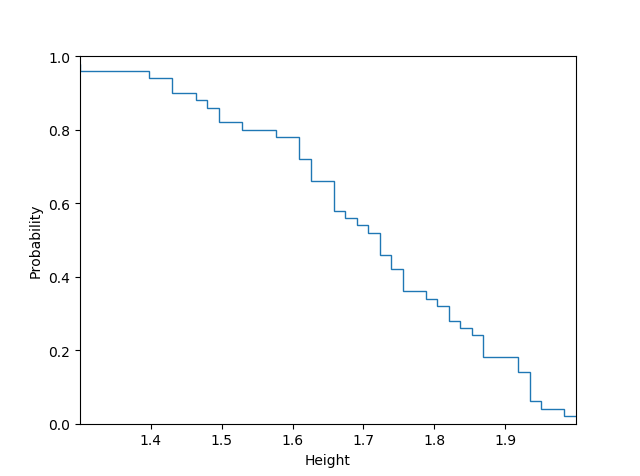由图可知，身高大于170厘米的概率大约为 $0.55$。
Quantile Funtion (PPF)，分位函数
又名 Percent Point Function，或者Inversed Cumulative Distribution Function，含义一目了然，就是CDF的反函数。以指数分布为例：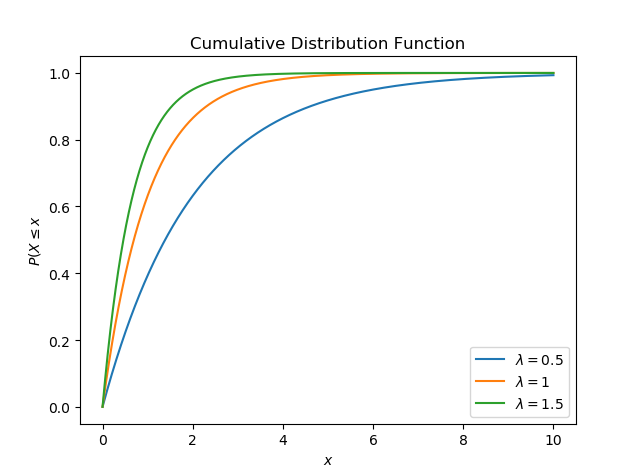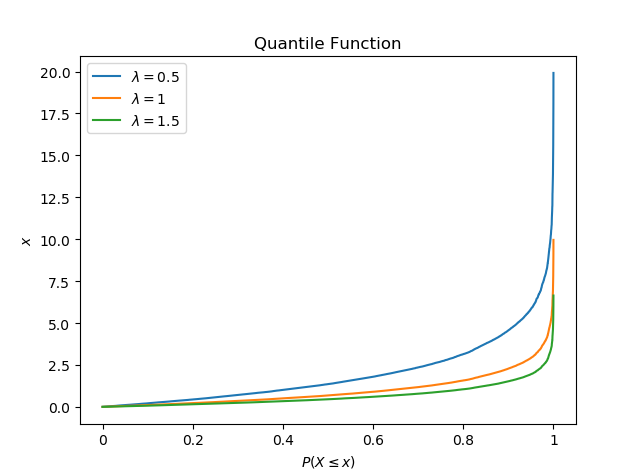比较两图可知，函数互为反函数。分位函数顾其名思其义，它的一大作用是分位点，以常见的四分位为例，对于 $\lambda = 1$ 指数分布，其四分位数分别为0.287、0.693、1.386，它们的含义是把样本从小到大排列，位于25%、50%、75%的数字 [13, 14]。也就是说，有25%的数字小于0.287，有25%的数字大于1.386。类似的还有二分位数和百分位数。分位函数广泛应用于统计学和蒙特卡洛方法 。
Dirac Delta Function，狄拉克 δ 函数
Exponential Distribution，指数分布

又称负指数分布，$X \sim \exp(\lambda)$，常用来描述事件发生的间隔时间，话不多说上公式：
$f(x;\lambda) = \begin{cases} \lambda e^{-\lambda x} & x \ge 0, \\ 0 & x < 0. \end{cases}$
概率密度函数（PDF）：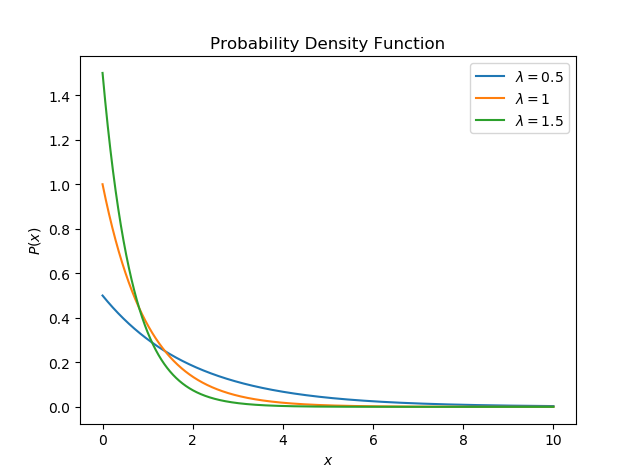$\lambda$ 越小表示单位时间内事件发生的次数越少。由图可知，随着 $x$ 的增加，事件发生的概率越来越小。
累积分布函数（CDF）：
$F(x;\lambda) = \begin{cases} 1 - e^{-\lambda x} & x \ge 0, \\ 0 & x < 0. \end{cases}$由指数分布的CDF图可知，随着时间的增加，事件发生的概率越来越大。
Heavy-tailed Distribution 重尾分布

重尾分布很有意思，先扔公式：
$\lim_{x \rarr \infty} e^{tx} \text{Pr}[X > x] = \infty \;\;\;\; \text{ for all } t > 0$
明天再写，告辞。
我回来了，继续。我们上面介绍了指数分布，它的概率密度函数的尾巴长长的，像老鼠尾巴 ，而且越往后，其值越小，$1/e^x$。重尾分布不一样，它越往后尾巴不一定越小。对于一个常规老鼠，它的尾巴占身体重量的比例是很少的，如果一个老鼠的尾巴超级长，它尾巴重量的占比将不断上升，最后甚至超过身体的重量。有没有想到那个著名的二八定律  ？它也叫帕累托分布（Pareto Distribution） ，对于这个整体的分布来说，不起眼的尾巴，其重要性甚至超过了本体，比如20%的人掌握了80%的财富等等，这个定律在自然界、社会、经济等方面都有体现 。回到原题，重尾分布的尾在哪并不重要，可以在右也可以在左，也可以左右都有，一般来说在右边。它的定义有一些分歧，一部分学者认为重尾分布的 power moments 是无限的，另外一部分学者认为重尾分布不具有一个有限的方差。重尾分布有三个重要的子类，（1）Fat-tailed distribution（2）Long-tailed distribution（3）Subexponential distribution，次指数分布。后面再提。
总的来说，当一个分布的尾巴很长，而且不是越长值越小，那么它就可以被称为重尾分布，其尾巴虽然看着不起眼，但在整体中占着主导地位。
在查找资料的过程中，我发现大家对重尾分布的理解有着很大的偏差，定义也不甚明确，下面主要用我自己的理解来说明。
角度一：转发数很高的微博占比很少，但是效果很出众。我们用正态分布和微博数据集分布的CCDF做一个对比，因为微博数据集的平均转发数为174.01， 所以正态分布的均值设为174.01，标准差设为150，共生成119,313个值：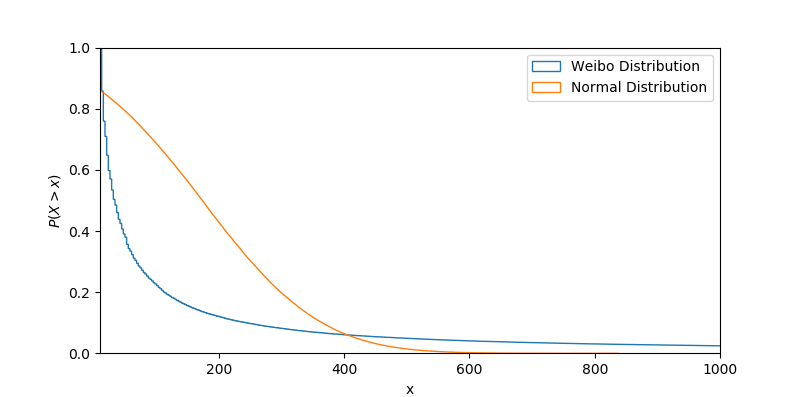很明显，微博数据集的尾巴要比正态分布厚很多，对于正态分布，概率衰减的非常快，而对于微博，随机变量 $X$ 大于某个 $x$ 的值的概率衰减的很慢。这意味着对于一条新的微博，它未来的转发数超过 $x$ 的概率要比正态分布大很多。如果微博转发数服从正态分布，那么对于一条新微博，它的转发数超过1,000的概率几乎为0，而微博的真实分布说明对于一条新微博，其转发数超过1,000的概率高达2.5%。
如果把所有微博按转发数排序从大到小，前20%的微博的转发数占了总转发数的84.65%：
角度二： 如果用转发数区间（单位为10）表示横坐标，用微博数表示纵坐标：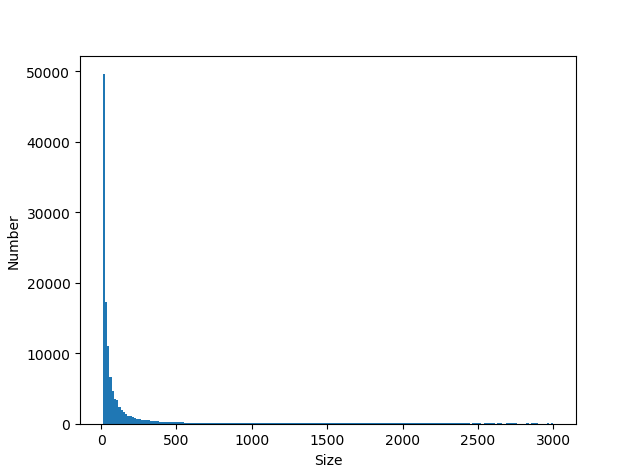由图可知，转发超过500的微博寥寥无几，大部分集中在 $[0, 200]$ 这个区间内，这个分布的尾巴在图里看起来毫无价值。但是尾巴中从 $[1000, \infty]$ 这个区间内的微博，贡献了84.65%的转发量。这个尾巴可算的上是重尾了。-_-
Long-tailed Distribution，长尾分布
扔公式先：
$\lim_{x \rarr \infty} \text{Pr} [X > x + t | X > x] = 1$
$\text{Pr}[X > x]$ 就是我们前面说过的CCDF。长尾分布与重尾分布相似但不同，长尾分布都是重尾分布，但重尾分布不一定是长尾分布。微博数据集虽然符合重尾分布，但是，根据常识我们知道，一条微博被转发1,000次和被转发2,000次的概率是不一样的，显然有
$\lim_{x \rarr \infty} \text{Pr} [X > 2000 | X > 1000] < 1$
长尾分布的潜在含义在于，如果 $X$ 超越了某个 $x$，它一定会超越更大的 $x$。
Subexponential Distribution，次指数分布
对于两个符合同一分布函数 $F$ 的随机变量 $X_1, X_2$，它们分布函数的卷积操作，定义为：
$\text{Pr}[X_1 + X_2 \le x] = F^{*2}(x) = \int_{-\infty}^{\infty} F(x - y)dF(y).$
可以推广到多个随机变量 $X_1, X_2, \dots, X_n$。尾分布函数为 $\bar{F}(x) = 1 - F(x)$.
如果分布 $F$ 的正半部分满足如下条件，则其符合次指数分布：
$\overline{F^{*n}} (x) \sim n \overline{F}(x) \;\;\;\; \text{as }x \rarr \infty$
其中 $n \ge 1$。次指数分布在各种风险模型中广泛应用，直观的理解就是，$n$ 个随机变量，它们的和超过某个 $x$ 的概率，和它们中最大的 $X_{max}$ 超过 $x$ 的概率等价。以保险行业为例，假设理赔金额满足次指数分布。如果你有10个保单，它们最终总的理赔金额超过10万的概率，和它们中某个金额最大的保单的单个理赔金额超过10万的概率等价。这就是说，另外9个保单的理赔金总和在后者面前几乎可以忽略不计：
$\text{Pr}[X_1+ X_2 + \dots + X_n > x] \sim \text{Pr}[max(X_1, X_2, \dots, X_n)] \;\;\;\; x \rarr \infty$
这也侧面说明，大部分的理赔金由少部分几个保单产生。容易证明，次指数分布都是长尾分布，长尾分布不一定是次指数分布。经济危机、地震灾害等都可视为次指数分布 。其在现实中的意义是极小概率发生的事件造成了极大影响 。
Fat-tailed Distribution，肥尾分布
肥尾分布一般指其尾部按幂率进行衰减，不过也不绝对，某些衰减的慢些的分布也被视为肥尾分布 [2, 3, 9]，例如对数正态分布、对数逻辑分布、帕累托分布等。
先扔公式：
$\text{Pr} [X > x] \sim x^{-\alpha} \;\;\;\; \text{as } x \rarr \infty, \;\;\;\; \alpha > 0$
当 $\alpha$ 不很大的时候，如果一个分布满足上述条件(即CCDF等价 $x^{-\alpha}$），则它可以称为肥尾分布。
说起 $\alpha$，我就想到美猴王头上的紧箍，今年春天，中美合拍，文体两开花，哦呸。还有一些概念涉及到重尾密度（Heavy-tailed Density）、尾部指数（Tail-index），我也没搞懂，有兴趣的可以自己看看。
Log-normal
Pareto Distribution
CDF公式投喂：
$\overline{F}(x) = \text{Pr}[X > x] = \begin{cases} 1-(\frac {x_m}{x})^a & x \ge x_m, \\ 0 & x < x_m. \end{cases}$
其中 $x_m$ 是 $X$ 的一个最小正值，$\alpha$ 是一个正参数。
扔完公式扔图：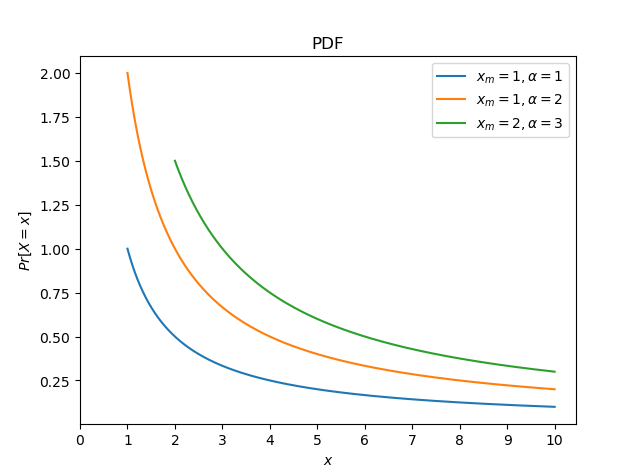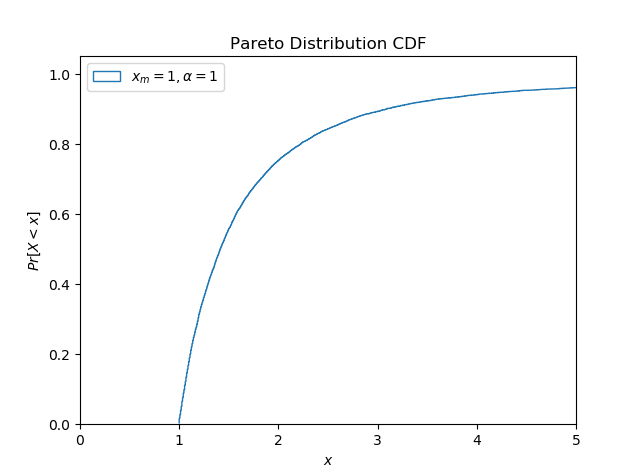帕累托分布一开始用来描述八二定律（叫二八定律也行），即20%的人掌握着80%的财富 ，其实这一条件是在尾部指数 $\alpha \approx 1.16$ 的时候取到的：Marginal Distribution
Marginal Joint Distribution
Multimodal Distribution
Bimodal Distribution
Multinomial Distribution
Power-law
Unimodal Distribution
Weibull Distribution
Zipfian Distribution (Zipf’s law)
Code

Cumulative Distribution Function
mu = 1.7
sigma = 0.2
n_bins = 50

np.random.seed(3197747)
height = np.random.normal(mu, sigma, n_bins)

plt.hist(height, n_bins, density=True, histtype='step', cumulative=True)

plt.axis([1.3, 2.0, 0, 1])
plt.xticks([1.4, 1.5, 1.6, 1.7, 1.8, 1.9])
plt.xlabel('Height')
plt.ylabel('Probability')

plt.show()

Quantile Funtion
lmbda_list = [0.5, 1, 1.5]
x = 1 - np.random.random(10000)

fig, ax = plt.subplots()

for lmbda in lmbda_list:
# y = [(1 - np.e ** (-1 * lmbda * x_)) for x_ in x[:]]
y = [-math.log(x_)/lmbda for x_ in x]
label = '$\lambda =$' + str(lmbda)
ax.plot(sorted(y), label=label)

plt.title('Quantile Function')

plt.xticks(np.arange(0, 10001, 2000), ('0', '0.2', '0.4', '0.6', '0.8',
'1'))
plt.xlabel('$P(X \leq x)$')
plt.ylabel('$x$')
plt.legend()
plt.show()

Exponential Distribution Function
# Probability Density Function
lmbda_list = [0.5, 1, 1.5]
x = np.arange(0, 10, 0.001)

fig, ax = plt.subplots()

for lmbda in lmbda_list:
y = [(lmbda * np.e ** (-1 * lmbda * x_)) for x_ in x[:]]
label = '$\lambda =$' + str(lmbda)
ax.plot(x, y, label=label)

plt.title('Probability Density Function')
plt.xlabel('$x$')
plt.ylabel('$P(x)$')
plt.legend()
plt.show()

# CDF
lmbda_list = [0.5, 1, 1.5]
x = np.arange(0, 12, 0.001)

fig, ax = plt.subplots()

for lmbda in lmbda_list:
y = [(1 - np.e ** (-1 * lmbda * x_)) for x_ in x[:]]
label = '$\lambda =$' + str(lmbda)
ax.plot(x, y, label=label)

plt.title('Cumulative Distribution Function')
plt.xlabel('$x$')
plt.ylabel('$P(X \leq x$')
plt.legend()
plt.show()

Pareto Distribution
# PDF
fig, ax = plt.subplots()

alpha = [1, 2, 3]
x_m = [1, 1, 2]
for i in range(len(alpha)):
x = np.arange(x_m[i], 10, 0.001)
y = [(alpha[i]*pow(x_, alpha[i])/(pow(x_, alpha[i]+1))) for x_ in x]
label = '$x_m = ' + str(x_m[i]) + ' ,\\alpha =$' + str(alpha[i])
ax.plot(x, y, label=label)

plt.xlabel('$x$')
plt.ylabel('$Pr[X = x]$')
plt.title('PDF')
plt.xticks(np.arange(0, 11))
plt.legend()
plt.show()

# CDF
fig, ax = plt.subplots()

alpha = [1, 2, 3]
x_m = [1, 1, 2]
for i in range(len(alpha)):
np.random.seed(3197747)
x = 1 - np.random.random(10000)
# reverse function
y = [(alpha[i] * pow(x_m[i], alpha[i]) / x_) ** (1 / (alpha[i] + 1))
for x_ in x]
label = '$x_m = ' + str(x_m[i]) + ' ,\\alpha =$' + str(alpha[i])

ax.hist(y, len(y), cumulative=True, density=True, histtype='step',
label=label)

plt.xlim(0, 5)
plt.xlabel('$x$')
plt.ylabel('$Pr[X < x]$')
plt.title('Pareto Distribution CDF')
plt.legend(loc=2)
plt.show()

Reference

Cumulative distribution function. (January 6, 2019). Retrieved from https://en.wikipedia.org/wiki/Cumulative_distribution_function
Heavy-tailed distribution. (December 18, 2018). Retrieved from https://en.wikipedia.org/wiki/Heavy-tailed_distribution
Fat-tailed distribution. (Octorber 12, 2018). Retrieved from https://en.wikipedia.org/wiki/Fat-tailed_distribution
Exponential distribution. (December 8, 2018). Retrieved from https://en.wikipedia.org/wiki/Exponential_distribution
Pareto distribution. (January 9, 2019). Retrieved from  https://en.wikipedia.org/wiki/Pareto_distribution
林建希. (2007). 关于次指数分布及其相关类的一个性质. Journal of Xiamen University (Nature Science), Retrieved from http://www.doc88.com/p-1963143584484.html
李芝棠. (January 26, 2019). Retrieved from https://wenku.baidu.com/view/208864738e9951e79a892705.html
二八定律. (January 26, 2019). Retrieved from https://baike.baidu.com/item/%E4%BA%8C%E5%85%AB%E5%AE%9A%E5%BE%8B/747076
dymodi. (January 8, 2017). 重尾分布，长尾分布，肥尾分布 和 随机游走 （Heavy-tailed, Long-tailed, Fat-tailed distribution and Random walk）. Retrieved from https://blog.csdn.net/dymodi/article/details/54231728
黄世宇. (December 26, 2016). 长尾分布，重尾分布(Heavy-tailed Distribution). Retrieved from https://www.cnblogs.com/huangshiyu13/p/6217180.html
西蒙斯. (August 18, 2018). 什么是肥尾效应？. Retrieved from http://www.zcaijing.com/ximengsi/105148.html
Cao, Q., Shen, H., Cen, K., Ouyang, W., & Cheng, X. (2017, November). DeepHawkes: Bridging the gap between prediction and understanding of information cascades. In Proceedings of the 2017 ACM on Conference on Information and Knowledge Management (pp. 1149-1158). ACM.
分位数. (August 14, 2018). Retrieved from https://baike.baidu.com/item/%E5%88%86%E4%BD%8D%E6%95%B0/10064158
Quantile function. (January 20, 2019). Retrieved from https://en.wikipedia.org/wiki/Quantile_function
Monte Carlo method. (January 14, 2019). Retrieved from https://en.wikipedia.org/wiki/Monte_Carlo_method


展开全文数学 统计学 Python
• 概率密度函数是概率论中的核心概念之一，用于描述连续型随机变量所服从的概率分布。 从随机事件说起 回忆我们在学习概率论时的经历，随机事件是第一个核心的概念，它定义为可能发生也可能不发生的事件，因此是否发生...
1. 概率函数
概率函数，就是用函数的形式来表达概率。
$p_i=P(X=a_i)(i=1,2,3,4,5,6)$
在这个函数里，自变量（X）是随机变量的取值，因变量（$p_i$）是取值的概率。这就叫啥，这叫用数学语言来表示自然现象！它就代表了每个取值的概率，所以顺理成章的它就叫做了X的概率函数。
从公式上来看，概率函数一次只能表示一个取值的概率。比如P（X=1）=1/6,这代表用概率函数的形式来表示，当随机变量取值为1的概率为1/6，一次只能代表一个随机变量的取值。
2. 概率分布
概率分布，就是概率的分布，这个概率分布还是讲概率的。我认为在理解这个概念时，关键不在于“概率”两个字，而在于“分布”这两个字。为了理解“分布”这个词，我们来看一张图。在很多教材中，这样的列表都被叫做离散型随机变量的“概率分布”。其实严格来说，它应该叫“离散型随机变量的值分布和值的概率分布列表”，这个名字虽然比“概率分布”长了点，但是对于我们这些笨学生来说，肯定好理解了很多。因为这个列表，上面是值，下面是这个取值相应取到的概率，而且这个列表把所有可能出现的情况全部都列出来了！
举个例子吧，一颗6面的骰子，有1，2，3，4，5，6这6个取值，每个取值取到的概率都为1/6。那么你说这个列表是不是这个骰子取值的”概率分布“？长得挺像的，上面是取值，下面是概率，这应该就是骰子取值的“概率分布”了吧！大错特错！少了一个最重要的条件！对于一颗骰子的取值来说，它列出的不是全部的取值，把6漏掉了!
这么一说你就应该明白概率分布是个什么鬼了吧。
3. 分布函数
说完概率分布，就该说说分布函数了。这个分布函数又是个简化版的东西！我真的很讨厌我们的教材中老是故弄玄虚，卖弄概念！你就老老实实的写成”概率分布函数“，让我们这些笨学生好理解一些不行吗？
看看下图中的分布律！这又是一个不统一叫法的丑恶典型！这里的分布律明明就是我们刚刚讲的“概率函数”，完全就是一个东西嘛！但是我知道很多教材就是叫分布律的。我们来看看图上的公式，其中的F(x)就代表概率分布函数啦。这个符号的右边是一个长的很像概率函数的公式，但是其中的等号变成了大于等于号的公式。你再往右看看，这是一个一个的概率函数的累加！发现概率分布函数的秘密了吗？它其实根本不是个新事物，它就是概率函数取值的累加结果！所以它又叫累积概率函数！其实，我觉得叫它累积概率函数还更好理解！！
概率函数和概率分布函数就像是一个硬币的两面，它们都只是描述概率的不同手段！
4. 概率密度函数
概率密度函数是概率论中的核心概念之一，用于描述连续型随机变量所服从的概率分布。
4.1 从随机事件说起
研究一个随机变量，不只是要看它能取哪些值，更重要的是它取各种值的概率如何！
回忆我们在学习概率论时的经历，随机事件是第一个核心的概念，它定义为可能发生也可能不发生的事件，因此是否发生具有随机性。例如，抛一枚硬币，可能正面朝上，也可能反面朝上，正面朝上或者反面朝上都是随机事件。掷骰子，1到6这6种点数都可能朝上，每种点数朝上，都是随机事件。4.2 整数集与实数集
高中时我们学过集合的概念，并且知道整数集是z，实数集是R。对于有限集，可以统计集合中元素的数量即集合的基数（cardinal number，也称为集合的势cardinality）。对于无限集，元素的个数显然是无穷大，但是，都是无穷大，能不能分个三六九等呢？
回忆微积分中的极限，对于下面的极限：虽然当x趋向于正无穷的时候，x和exp(x)都是无穷大，但它们是有级别的，在exp(x)面前，x是小巫见老巫。
同样的，对于整数集和实数集，也是有级别大小的。任意两个整数之间，如1与2之间，都密密麻麻的分布着无穷多个实数，而且，只要两个实数不相等，不管它们之间有多靠近，如0.0000001和0.0000002，在它们之间还有无穷多个实数。在数轴上，整数是离散的，而实数则是连续的，密密麻麻的布满整个数轴。因此，实数集的元素个数显然比整数要高一个级别。
4.3 随机变量
变量是我们再熟悉不过的概念，它是指一个变化的量，可以取各种不同的值。随机变量可以看做是关联了概率值的变量，即变量取每个值有一定的概率。例如，你买彩票，最后的中奖金额x就是一个随机变量，它的取值有3种情况，以0.9的概率中0元，0.09的概率中100元，0.01的概率中1000元。变量的取值来自一个集合，可以是有限集，也可以是无限集。对于无限集，可以是离散的，也可以是连续的，前者对应于整数集，后者对应于实数集。
4.3.1 离散型随机变量
随机变量是取值有多种可能并且取每个值都有一个概率的变量。它分为离散型和连续型两种，离散型随机变量的取值为有限个或者无限可列个（整数集是典型的无限可列），连续型随机变量的取值为无限不可列个（实数集是典型的无限不可列）。4.3.2 连续型随机变量
把分布表推广到无限情况，就可以得到连续型随机变量的概率密度函数。此时，随机变量取每个具体的值的概率为0，但在落在每一点处的概率是有相对大小的，描述这个概念的，就是概率密度函数。你可以把这个想象成一个实心物体，在每一点处质量为0，但是有密度，即有相对质量大小。在概率论和统计学中，拉普拉斯是一种连续概率分布。由于它可以看做是俩个不同位置的指数分布背靠背拼在一起，所以它也叫做双指数分布。如果随机变量的概率密度函数分布为：那么他就是拉普拉斯分布。u为位置参数，b>0是尺度参数。与正态分布相比，正态分布是用相对于u平均值的差的平方来表示，而拉普拉斯概率密度用相对于差的绝对值来表示。因此，拉普拉斯的尾部比正态分布更加平坦。概率密度函数用数学公式表示就是一个定积分的函数，定积分在数学中是用来求面积的，而在这里，你就把概率表示为面积即可！左边是F(x)连续型随机变量分布函数画出的图形，右边是f(x)连续型随机变量的概率密度函数画出的图像，它们之间的关系就是，概率密度函数是分布函数的导函数。
两张图一对比，你就会发现，如果用右图中的面积来表示概率，利用图形就能很清楚的看出，哪些取值的概率更大！这样看起来是不是特别直观，特别爽！！所以，我们在表示连续型随机变量的概率时，用f(x)概率密度函数来表示，是非常好的！
但是，可能读者会有这样的问题：
Q：概率密度函数在某一点的值有什么意义？
A：比较容易理解的意义，某点的 概率密度函数 即为 概率在该点的变化率(或导数)。很容易误以为 该点概率密度值 为 概率值.
比如: 距离(概率)和速度(概率密度)的关系.某一点的速度, 不能以为是某一点的距离，没意义,因为距离是从XX到XX的概念，所以, 概率也需要有个区间.
这个区间可以是x的邻域（可以无限趋近于0）。对x邻域内的f（x）进行积分，可以求得这个邻域的面积，就代表了这个邻域所代表这个事件发生的概率。
4.4 期望E(X)与方差Var(X)
随机变量(Random Variable)X是一个映射，把随机试验的结果与实数建立起了一一对应的关系。而期望与方差是随机变量的两个重要的数字特这。
期望(Expectation, or expected value)是度量一个随机变量取值的集中位置或平均水平的最基本的数字特征；
方差(Variance)是表示随机变量取值的分散性的一个数字特征。 方差越大，说明随机变量的取值分布越不均匀，变化性越强；方差越小，说明随机变量的取值越趋近于均值，即期望值。4.4.1 期望和方差的运算性质
4.4.1.1 期望运算性质4.4.1.2 方差的运算性质4.4.1.3 期望与方差的联系4.4.2 协方差4.4.2.1 协方差的运算性质4.4.3 相关系数
4.4.3.1 定义
相关系数通过方差和协方差定义。两个随机变量的相关系数被定义为：4.4.3.2 性质
1、有界性
相关系数的取值范围为-1到1，其可以看成是无量纲的协方差。
2、统计意义
值越接近1，说明两个变量正相关性（线性）越强，越接近-1，说明负相关性越强，当为0时表示两个变量没有相关性。5. 常见概率分布
5.1 均匀分布（Uniform Distribution）5.2 伯努利分布（Bernoulli Distribution）5.3 二项分布（Binomial Distribution）
二项分布(Binomial distribution)是n重伯努利试验成功次数的离散概率分布。从定义可以看出，伯努利分布是二项分布在n=1时的特例5.4 负二项分布（Negative Binomial Distribution）5.5 几何分布（Geometric Distribution）
假定我们有一系列伯努利试验，其中每一个的成功概率为$p$，失败概率为$q=1-p$。在获得一次成功前要进行多次试验？
注意，这里的随机变量的概率分布就是一种几何分布。具体如下：几何分布的概率分布图如下，见之会有更形象地认知。为什么单独把几何分布和二项分布单独列出，一方面其代表的概率试验的普适性，另一方面其期望和方差都是有特殊技巧。其实有意思的是，这里面的求解过程；但是本文不具体涉及了。因为像几何分布和二项分布这种可能要多写几章，当然是否连续写就不知道了。本着实用主义来。
一般简单地肯定在前面讲，复杂一些得也更有意思一些的肯定是在后面，比如二项分布明显就在几何分布后面了。
不同于几何分布描述的运行到第几次才成功，二项分布描述是的N次试验里有多少次成功。具体如下：5.6 超几何分布（Hypergeometric Distibution）5.7 正态/高斯分布 (Normal / Gaussian Distribution)
正态分布是很多应用中的合理选择。如果某个随机变量取值范围是实数，且对它的概率分布一无所知，通常会假设它服从正态分布。有两个原因支持这一选择：

建模的任务的真实分布通常都确实接近正态分布。中心极限定理表明，多个独立随机变量的和近似正态分布。
在具有相同方差的所有可能的概率分布中，正态分布的熵最大（即不确定性最大）。5.7.1 一维正态分布5.7.2 多维正态分布5.8 拉普拉斯分布5.9 泊松分布（Poisson Distribution）5.10 指数分布5.11 伽马分布5.12 贝塔分布5.13 狄拉克分布5.14 多项式分布与狄里克雷分布
多项式分布(Multinomial Distribution)是二项式分布的推广。二项式做n次伯努利实验，规定了每次试验的结果只有两个，如果现在还是做n次试验，只不过每次试验的结果可以有多m个，且m个结果发生的概率互斥且和为1，则发生其中一个结果X次的概率就是多项式分布。
扔骰子是典型的多项式分布。扔骰子，不同于扔硬币，骰子有6个面对应6个不同的点数，这样单次每个点数朝上的概率都是1/6（对应p1~p6，它们的值不一定都是1/6，只要和为1且互斥即可，比如一个形状不规则的骰子）,重复扔n次，如果问有k次都是点数6朝上的概率就是5.15 混合概率分布5.16 总结https://www.jianshu.com/p/b570b1ba92bb
https://zhuanlan.zhihu.com/p/48140593
https://www.bookstack.cn/read/huaxiaozhuan-ai/spilt.4.6f06ed449f5ed789.md
https://zhuanlan.zhihu.com/p/94181395
https://zhuanlan.zhihu.com/p/64859161
https://blog.csdn.net/touristman5/article/details/56281887


展开全文• 常见概率分布及在R中的应用 转载 ：http://eyejava.iteye.com/blog/324189 常见概率分布及在R中的应用 博客分类：  BI QtF#PHP    R提供工具来计算累计分布函数p(cummulative ...R
• 摘要： 　1.常见离散变量的分布 ...3.1 在贝叶斯概率理论，如果后验概率和先验概率满足同样的分布律，那么先验分布和后验分布被叫做共轭分布 3.2 证明Beta分布和二项分布互为共轭分布： ...
• 生活中的很多事情潜在的都符合某种规律。例如：反复抛掷一枚均匀的硬币，出现正面和反面的机会是差不多的；我们认识的人里面特别高或者特别矮的都不多，大部分人的身高都在一个比较接近的范围内。概率论通过概率分布...
• 定义：n次独立重复伯努利试验，设每次试验事件A发生的概率为p，用X表示n重伯努利试验事件A发生次数，则X可能取值为0，1，…，n,且对每一个k（0≤k≤n）,事件{X=k}即为“n次试验事件A恰好发生k次”，随
• https://blog.csdn.net/qq_32806793/article/details/99059670 感谢大佬通俗解释。机器学习
• 2 离散型随机变量的概率分布 2.1二项分布 2.2超几何分布 2.2.1 概念 2.2.2 举例 2.3泊松分布 3 连续型随机变量的概率分布 3.1均匀分布 3.1.1 概念 3.2 正态分布 3.2.1 概念 3.3指数分布 3.3.1 概念 ...概率论与数理统计
• 连续分布 1.均匀分布 class pymc3.distributions.continuous.Uniform（lower = 0，...均匀分布的概率密度函数为： import numpy as np import matplotlib.pyplot as plt plt.style.use('seaborn-darkgrid...PyMC3
• 每个概率分布都有一个概率分布函数，输入样本值概率分布函数输出当前这个样本属于某个类的概率。而任何概率分布函数都会存在未知参数，不同数据要取不同参数。比如逻辑回归，它就认为概率分布函数长像这样，输入...
• 最近几日又把概率导论拿出来瞅瞅，重要公式自己去推导，这些需要重点记，仍然需要记下来。 1累积分布函数 2伯努利随机变量 3 二项随机变量 4 几何随机变量 5 柏松随机变量 ...
• 每次我们开始探索新数据集时，我们首先需要进行探索性数据分析（EDA），以了解某些特征的概率分布是什么。如果我们能够了解数据分布中是否存在特定模式，则可以量身定制最适合我们机器学习模型。这样，我们将...
• distribution），是一个在数学、物理及工程等领域都非常重要的概率分布，在统计学许多方面有着重大影响力。 若随机变量X服从一个数学期望为μ、标准方差为σ2高斯分布，记为： X∼N(μ,σ2), 则其概率密度...统计学 伽玛分布
• 每次我们开始探索新数据集时，我们首先需要进行探索性数据分析（EDA），以了解某些特征的概率分布是什么。如果我们能够了解数据分布中是否存在特定模式，则可以量身定制最适合我们机器学习模型。这样，我们将...
• 作者：Pier Paolo Ippolito@南安普敦大学 编译：机器学习算法与Python实战（微信公众号：tjxj666） ...拥有良好统计背景对于数据科学家日常工作可能会大有裨益。每次我们开始探索新数据集时...
• 1.两点分布 即只先进行一次伯努利试验，该事件发生的概率为p，不发生的概率为1-p。这是一个最简单的分布...在n次伯努利实验，第k次实验才得到第一次成功的概率分布。其中：P(k) = (1-p)^(k-1)*p 期望：E=1/p 方差：D
• 设在一个试验事件 A 发生的概率是 ppp，则独立重复 nnn 次试验后，事件 A 发生 iii 次的概率为 pi=B(n,p)=(ni)pi(1−p)n−i,i=0,1,...,np_i = B(n, p) = \left(\begin{matrix} n \\ i \end{matrix}\right)p^i(1-p...
• distribution）是n个独立是/非试验成功次数离散概率分布，其中每次试验成功概率为p。这样单次成功/失败试验又称为伯努利试验。实际上，当n= 1时，二项分布就是伯努利分布。二项分布是显著性差异二项...
• 事件A在某次试验发生的概率稳定计为pp，但A要么发生要么不发生，随机变量XX，单次试验A发生记为1，没有发生记为0，则P(X=1)=p,P(X=0)=1−pP(X=1)=p,P(X=0)=1-p，也可以统一成这个公式： f(x|p)=px(1−p)1−x,x=......